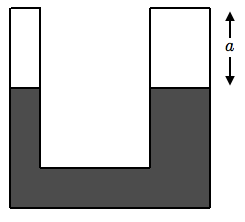# U tubes are tricky!A U-tube is filled with mercury as shown below, leaving a distance $a$ without filling. The cross section of the right hand of the tube is twice the cross section of the left one. Water is then poured water on the right hand of the tube until the water level reaches the top of the right side. If the level of mercury in the left side of the tube rises a distance

$H=\frac{b}{c}a$

where $b$ and $c$ are positive co-prime integers, find the value of $b+c$.

Take the density of mercury to be 13.6 times the density of water.

###### This problem is not original. It is taken from this year's Second Round of the Colombian Physics Olympiad.
×

Problem Loading...

Note Loading...

Set Loading...